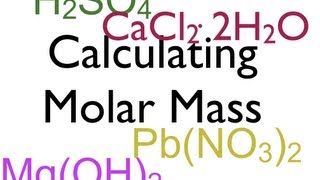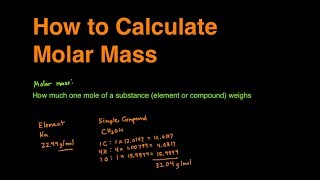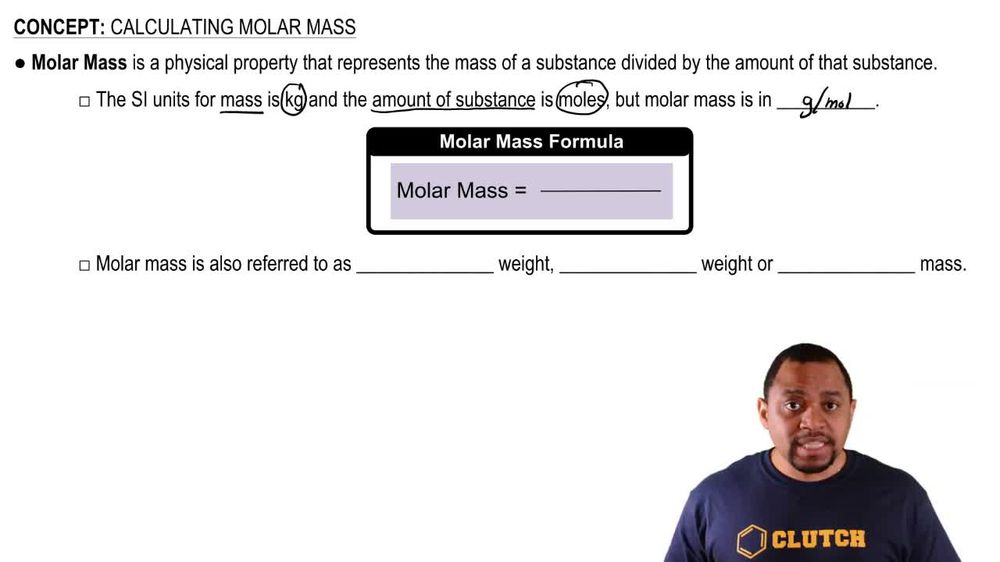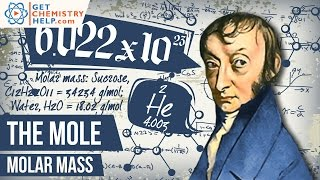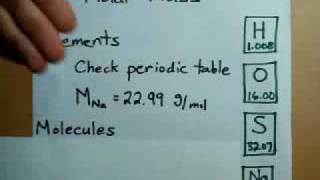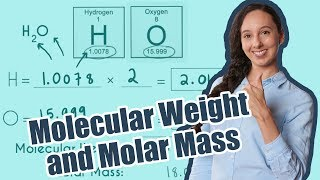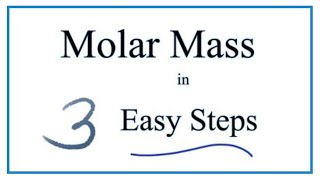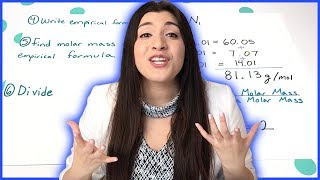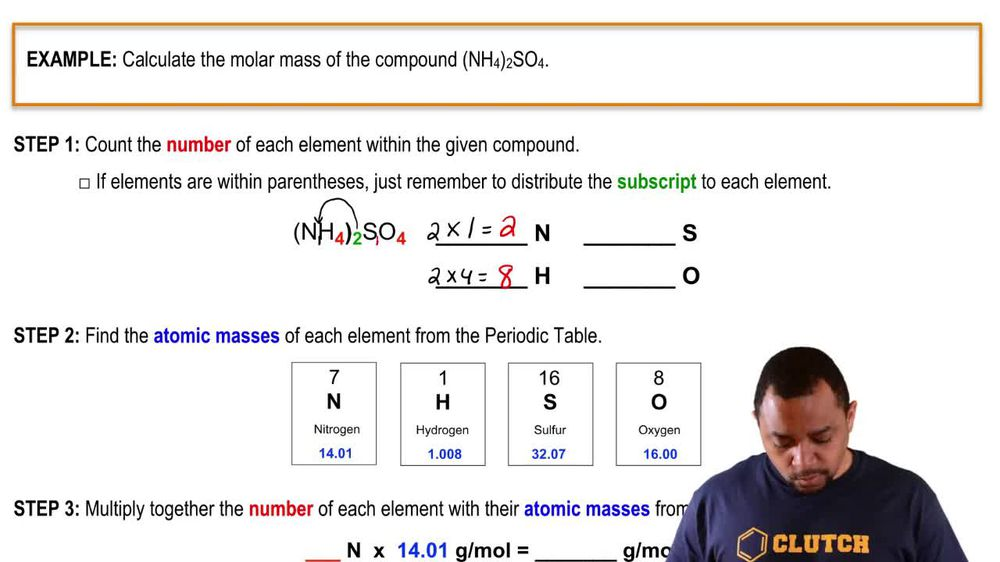Start typing, then use the up and down arrows to select an option from the list.
1. 2. Atoms & Elements2. Calculating Molar Mass
Problem

# What are the molecular (formula) weights of the following substances? (a) Hg2Cl2 (calomel, used at one time as a bowel purgative)

Relevant Solution2m
Play a video:
Hey everyone. Let's go ahead and practice calculating our molar masses. So starting off with sodium bromate, we have one in sodium and we're going to take each element and count the number of moles each one has and multiply it by its atomic mass. So for sodium we have one of sodium and we're going to multiply this by 22.99 g per mole. This will get us to 22.99 g per mole. Moving on to bro, mean we have one of bromine as well and we're going to multiply this by 79.904. This will get us to a value of 79.904. And lastly we have three of oxygen and we're going to multiply this by 16.0 This will get us to a value of 48. And when we take the total of this, We end up with a molar mass of 150.89 grams per mole. And make sure you have at least five significant figures per our question. Now, moving on to our next compound, we have 13 carbons And we're going to multiply this by 12.01 g per mole, Which will get us to 156.13. Moving on to hydrogen, we have six of hydrogen and we're going to multiply this by 1. g per mole, which will get us to 6.6. And lastly looking at our chlorine, we have four of chlorine, which we're going to multiply by 35.45 grams per mole, Which will get us a total of 141.8. So getting the total of all these, We end up with a molar mass of 0.99 grams per mole. So this is going to be our answers for both halogen containing compounds. So I hope that made sense and let us know if you have any questions.